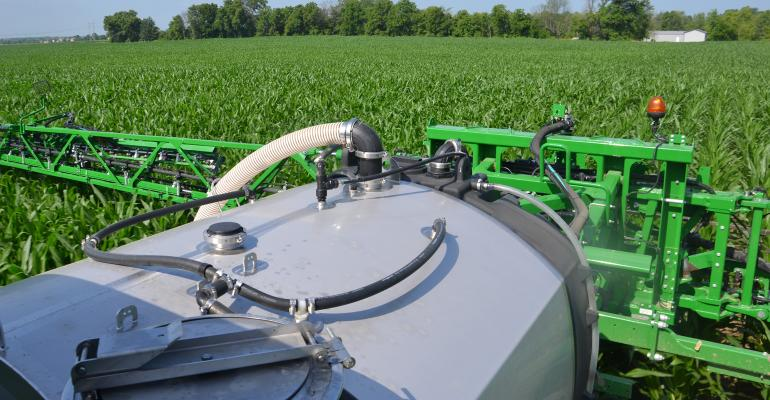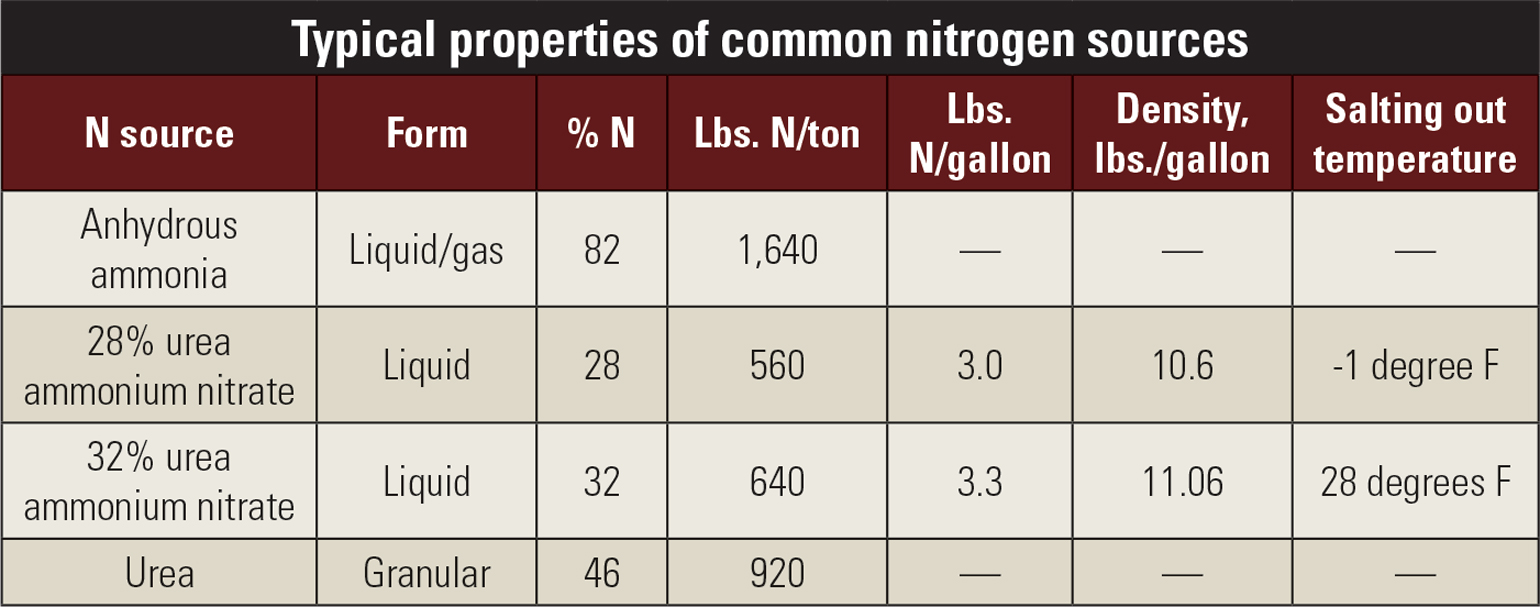# Basic calculations for nitrogen fertilizersBasic calculations for nitrogen fertilizers

Know how many pounds of actual nitrogen you’re applying with any source.REAL RATE: If you’re applying 30 gallons of 28% N in this pass, how many pounds of N are you applying? Based on the table below, you would be applying 90 pounds per acre.Tom J. Bechman

Two farming partners reached a revelation. They applied about 20 pounds of nitrogen per acre less in 2022 than they thought. Why? Because the partner determining rates made a basic error in converting gallons of liquid nitrogen to pounds of nitrogen per acre.

Tight margins don’t allow room for error. This new series will focus on basic key questions about fertilizer that deserve solid, straightforward answers. Jim Camberato, Purdue Extension soil fertility specialist, provides answers in this inaugural article. To submit questions, email [email protected] or mail them to 599 N., 100 W., Franklin, IN 46131.

How can you calculate pounds of nitrogen supplied from gallons of liquid fertilizer? The percentage of the nutrient and the density of the liquid in pounds per gallon are the only two pieces of information needed to calculate how many pounds of nutrient are in a gallon of liquid fertilizer. Refer to the table to see percentages of nitrogen and density in 28% N and 32% N.

For example: Consider 28% urea ammonium nitrate. It is 28% N and has a density of 10.6 pounds per gallon. To find the amount of actual N per gallon of 28%, simply multiply 28 by 10.6, then divide by 100 to get pounds per gallon of 28%. The math is: 28 x 10.6 / 100 = 2.97. For practical purposes, round it off to 3 pounds of N per gallon, as noted in the table.So, if I apply 20 gallons of 28% liquid N, how many pounds am I applying? It’s straightforward math. If you apply 20 gallons times 3 pounds per gallon, you applied 60 pounds per acre. Technically, it’s 59.4 pounds of actual N, but 60 is likely close enough for your purposes.

I apply 32% liquid N. What does the math look like for me? The density is 11.06 pounds per gallon. So, the math is: 32 x 11.06 / 100 = 3.54, or about 3.5 pounds per gallon. If you applied the same 20 gallons with 32% N, you would apply about 70 pounds per acre.

Subscribe to receive top agriculture news
Be informed daily with these free e-newsletters

You May Also Like

Aug 29 - Aug 31, 2023
Farm Progress Show annually hosts more than 600 exhibitors displaying new farm equipment, tractors, combines and farm implements; seed and crop protection products; and many additional farm supplies and services.